Math Courses / Course / Chapter

## Test Prep Plan - Take a practice test

Take this practice test to check your existing knowledge of the course material. We'll review your answers and create a Test Prep Plan for you based on your results.
How Test Prep Plans work
1
Test your existing knowledge.
2View your test results
Based on your results, we'll create a customized Test Prep Plan just for you!
3Study smarter
Study more effectively: skip concepts you already know and focus on what you still need to learn.

# Limits of Sequences & Functions Chapter Exam

Exam Instructions:

Choose your answers to the questions and click 'Next' to see the next set of questions. You can skip questions if you would like and come back to them later with the "Go To First Skipped Question" button. When you have completed the practice exam, a green submit button will appear. Click it to see your results. Good luck!

Answered 0 of 30

### Page 1

#### Question 4 4. Which of the following is true?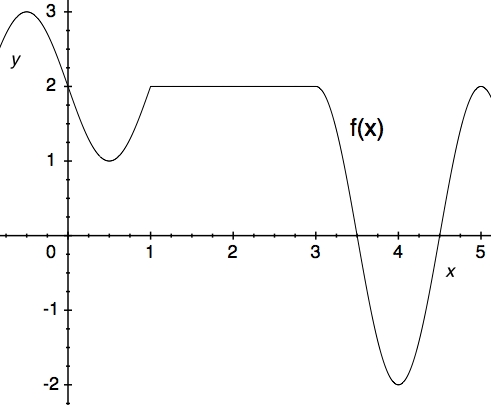#### Question 5 5. Evaluate the limit below.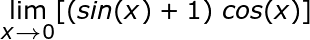### Page 2

#### Question 6 6. Evaluate the limit below.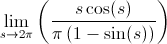#### Question 7 7. Use the given graph and formula to solve for z.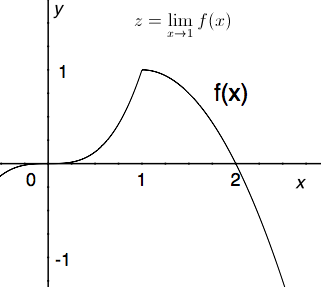#### Question 8 8. Use the given formula to solve for z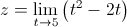#### Question 9 9. Use the graph to solve for z.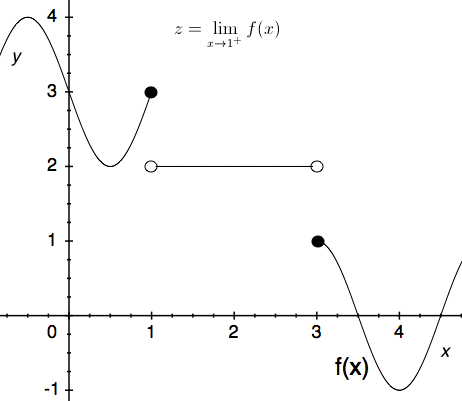### Page 3

#### Question 11 11. What are the regions of continuity given the following?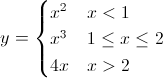### Page 4

#### Question 17 17. Consider the function below. According to the intermediate value theorem, is there a solution to f(x) = 0 for a value of x between -5 and 5?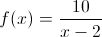#### Question 18 18. Consider the function f(x) below. Considering the intermediate value theorem and the vertical asymptote at x=0, is the following statement true or false? For any number C greater than 0, there is a positive value of x that satisfies the equation f(x)=C.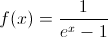#### Question 19 19. Using the squeeze theorem, what is z?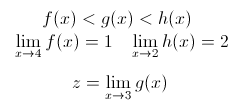#### Question 20 20. Using the squeeze theorem, what is z?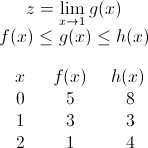### Page 5

#### Question 25 25. How many discontinuities are in this function?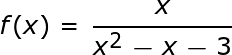### Page 6

#### Question 26 26. Use the graph to solve for z.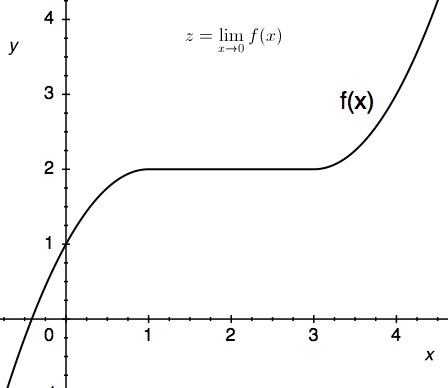#### Question 27 27. The expression below can also be written as: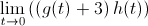#### Question 28 28. Solve for the limit: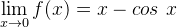#### Question 30 30. What are the regions of continuity for y defined as?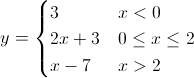#### Limits of Sequences & Functions Chapter Exam Instructions

Choose your answers to the questions and click 'Next' to see the next set of questions. You can skip questions if you would like and come back to them later with the "Go To First Skipped Question" button. When you have completed the practice exam, a green submit button will appear. Click it to see your results. Good luck!

Math Courses / Course / Chapter

Support